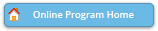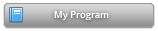#### Abstract Details

 Activity Number: 190 - Contributed Poster Presentations: Section on Statistics and the Environment Type: Contributed Date/Time: Monday, July 29, 2019 : 10:30 AM to 12:20 PM Sponsor: Section on Statistics and the Environment Abstract #305166 Title: Tail Dependence of Normal Mean-Variance Mixtures Author(s): Zhongwei Zhang* and Raphaël Huser Companies: and King Abdullah University of Science and Technology Keywords: tail dependence; normal mean-variance mixture; generalized hyperbolic distribution; spatio-temporal extremes Abstract: A random vector $X$ is a normal mean-variance mixture provided $X$, for a given $W \geq 0$, follows a multivariate normal distribution with mean vector $\mu + \beta W$ and covariance matrix $W\Sigma$, and provided $W$ follows a distribution $F$ on $[0,\infty)$. This provides a very flexible family class and by properly choosing the parameters and mixing distribution, many well-known multivariate distributions can be obtained. A prominent example is the generalized hyperbolic (GH) distribution, when the mixing distribution is generalized inverse Gaussian. In this work, we study the tail dependence properties of the GH distribution and derive its coefficient of tail dependence, which controls the joint tail decay rate. We also investigate the tail dependence structure of normal mean-variance mixtures without assuming specific mixing distributions. That is, the relation between the tail behavior of the normal mean-variance mixture and its mixing distribution is deduced. Based on this result, a spatio-temporal model for extremes is proposed.

Authors who are presenting talks have a * after their name.